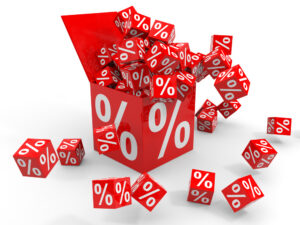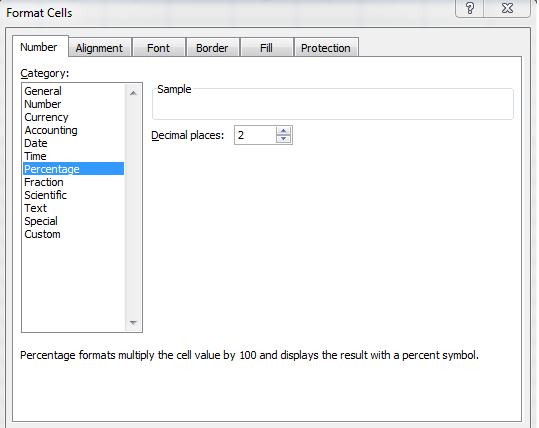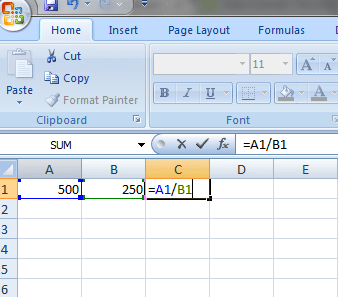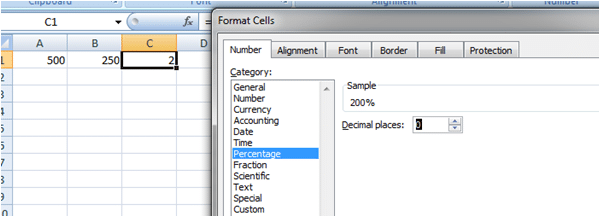Say you’ve been offered an easy payment option. You can something that costs \$5000 and pay it in 12 easy instalments of \$600 each month. Is that a good deal or what? But how much are you really paying back? What is the real interest rate you’re being charged?

Or say you’ve finally found a discount coupon for 22% off on iPhone 5. Is that a good deal? How much will you still have to shell out?

If you’re like most of us, you mostly can’t do that math in your head. Even trying it out on a calculator may be too taxing. Fear not, Excel can come to your rescue! If you’re new to Excel, we recommend you to try our beginners course on Excel before moving on. In this article we’re going to show you how you can use Excel percentage formula for tricky situations like there.

Tip: This course shows some other neat ways to make Excel do the work for you, and you may want to check it out later.

## Percentage Basics

Percent means “per 100.” If you remember from high school math class, when you’re calculating a percentage, you divide the numerator by the denominator and multiply the result by 100. It simply means what part of hundred, does your numerator amount to.  It’s commonly used to calculate grades on a test score, sales data, or determine the percentage change in your business turn over. The simple formula for percentage is

Percentage= (Numerator/Denominator) *100

## The Format for Percentage in Excel

MS Excel has an option to format each cell. You can either format the enter range of cells or non-adjacent cells. The cells can have various formats such as currencies, fractions or percentages. When a cell is formatted as percentage values, it displays the percent sign, and any values entered in that cell(s) are automatically converted in the percentage format. If you want to convert any value into a percentage, just right click on it and select “Format Cells”. In the window that opens up, select “Percentage” like we’ve shown in this screenshot.If you want to format the entire column, just select the entire column. If you want to format non-adjacent cells, hold down the “CTRL” key and select the cells. You can look up this course for other awesome tips and tricks to using Excel like a Pro.

## How to calculate percentages in Excel

1. Open an Excel worksheet and enter a value which corresponds to an amount in one cell. This will be our Numerator. In another cell, enter “the total” value. This will be our denominator
2. Highlight a blank cell where you want to put the calculated percentage value.
3. To get the fractional value, in the blank cell you have to enter “= Cell1/Cell2.” For example, if you have values in A1 and B1, and you want the result in C1, go to C and type in the formula “= A1/B1.”1. Now  you want to format the C1 cell into percentage. Right click and select “Format Cells”.
2. Go to “Number” tab and Under “Category” Choose “Percentage.”Note that the percentage formula, rather division, will work only with Numerical values. If cell A1 and B1 are not numbers, Excel will show “#VALUE” in that cell with an error “A value used in the formula is of the wrong data type”.

## How to Get Percentages with Decimals

Normally you’d want to deal with percentages in integer ie whole numbers. But if you’re dealing with high precision data, you may want to use decimal places as well. Here’s how you can do that

1. Go to “Number” tab and Under “Category” Choose “Percentage.”
2. If you want to display the decimal places, on the right hand side you choose “Decimal places” drop down box. By default, its set to 2, which means by default the percentage will have up to two decimal places. You can increase or decrease the decimal places.
3. Click “Ok” to confirm the change.## How to Calculate Percentage change using a Simple Single Step Formula

If you want to subtract a percent from a number, say for an example “a 40% loss from a principal of \$60”,  here’s how you can do it with simple one step formula. Let’s take a look at it.

1. In your Excel worksheet, type the principal or  main value in cell A5. For example \$60.
1. In the adjacent cell enter the percentage you want to reduce it by –  for example, in cell A6 enter 40%.
1. Place your cursor on the cell, say cell A7 where you want the answer to be displayed. Here enter the formula “= A5-(A5*A6).” Here your answer will be 36. That is 40% of 60 is 24. 60 minus 24 equal to 36.

You can use the same method to calculate percentage increase as well.

This method is most often used by business owners to evaluate the gross margins, cost, total revenue and other financial ratios. You can try out this course to learn other Excel skills for Business owners.

## Calculate the difference between two numbers as a percentage using ABS Function

Let’s say you want to calculate the percentage change in your expenditure over two months. A simple way to do this is with the e Absolute function (Abs(). )

1. Suppose you have two columns – November expenditure in cell A1 and December expenditure cell B1, with values \$2500 and \$3000 respectively.
2. To find the percentage difference between the expenditure of two months use the formula given below
3. “= (B1-A1)/ABS (A1) “

This works out to (3000-2500)/ABS (2500) , which is 0.2. So your percentage increase in expenditure is 20%

Now that we’ve walked you through the basics of how to use percentage formula in Excel, can you go figure out whether that \$600 deal was good for you; and how much will you still need to shell out for that iPhone5? Leave your answers in the comment below. Oh, and if you ever need a primer to refresh your excel skills, just go look up this course on Excel 2013.

Page Last Updated: February 2014

### Top courses in Excel

Microsoft Excel: Data Analysis with Excel Pivot Tables
Maven Analytics, Chris Dutton
4.7 (57,239)
Bestseller
Microsoft Excel: Data Visualization, Excel Charts & Graphs
Maven Analytics, Chris Dutton
4.6 (44,411)
Bestseller
Microsoft Excel Pro Tips: Go from Beginner to Advanced Excel
Maven Analytics, Chris Dutton
4.7 (5,144)
Bestseller
Microsoft Excel - Excel from Beginner to Advanced
Kyle Pew, Office Newb
4.7 (409,869)
Bestseller
Microsoft Excel - Excel from Beginner to Advanced 2023
Warrick Klimaytys
4.6 (9,233)
Bestseller
Complete Excel Megacourse: Beginner to Expert
Creativity Unleashed
4.5 (370)
Microsoft Excel for Construction Management
James Gedney-Higham
4.5 (482)
Bestseller